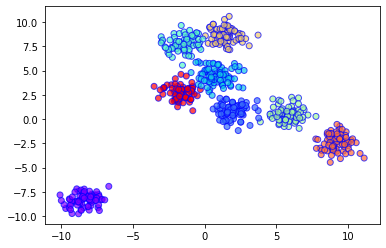# ML | BIRCH Clustering

Clustering algorithms like K-means clustering do not perform clustering very efficiently and it is difficult to process large datasets with a limited amount of resources (like memory or a slower CPU). So, regular clustering algorithms do not scale well in terms of running time and quality as the size of the dataset increases. This is where BIRCH clustering comes in.

Balanced Iterative Reducing and Clustering using Hierarchies (BIRCH) is a clustering algorithm that can cluster large datasets by first generating a small and compact summary of the the large dataset that retains as much information as possible. This smaller summary is then clustered instead of clustering the larger dataset.

BIRCH is often used to complement other clustering algorithms by creating a summary of the dataset that the other clustering algorithm can now use. However, BIRCH has one major drawback – it can only process metric attributes. A metric attribute is any attribute whose values can be represented in Euclidean space i.e., no categorical attributes should be present.

Before we implement BIRCH, we must understand two important terms: Clustering Feature (CF) and CF – Tree
Clustering Feature (CF):
BIRCH summarizes large datasets into smaller, dense regions called Clustering Feature (CF) entries. Formally, a Clustering Feature entry is defined as an ordered triple, (N, LS, SS) where ‘N’ is the number of data points in the cluster, ‘LS’ is the linear sum of the data points and ‘SS’ is the squared sum of the data points in the cluster. It is possible for a CF entry to be composed of other CF entries.

CF Tree:
The CF tree is the actual compact representation that we have been speaking of so far. A CF tree is a tree where each leaf node contains a sub-cluster. Every entry in a CF tree contains a pointer to a child node and a CF entry made up of the sum of CF entries in the child nodes. There is a maximum number of entries in each leaf node. This maximum number is called the threshold. We will learn more about what this threshold value is.

Parameters of BIRCH Algorithm :

• threshold :  threshold is the maximum number of data points a sub-cluster in the leaf node of the CF tree can hold.
• branching_factor : This parameter specifies the maximum number of CF sub-clusters in each node (internal node).
• n_clusters : The number of clusters to be returned after the entire BIRCH algorithm is complete i.e., number of clusters after the final clustering step. If set to None, the final clustering step is not performed and intermediate clusters are returned.

Implementation of BIRCH in Python:
For the sake of this example, we will generate a dataset for clustering using scikit-learn’s `make_blobs()` method. To learn more about make_blobs(), you can refer to the link below: https://scikit-learn.org/stable/modules/generated/sklearn.datasets.make_blobs.html

Code: To create 8 clusters with 600 randomly generated samples and then plotting the results in a scatter plot.

 `# Import required libraries and modules ` `import` `matplotlib.pyplot as plt ` `from` `sklearn.datasets.samples_generator ``import` `make_blobs ` `from` `sklearn.cluster ``import` `Birch ` ` `  `# Generating 600 samples using make_blobs ` `dataset, clusters ``=` `make_blobs(n_samples ``=` `600``, centers ``=` `8``, cluster_std ``=` `0.75``, random_state ``=` `0``) ` ` `  `# Creating the BIRCH clustering model ` `model ``=` `Birch(branching_factor ``=` `50``, n_clusters ``=` `None``, threshold ``=` `1.5``) ` ` `  `# Fit the data (Training) ` `model.fit(dataset) ` ` `  `# Predict the same data ` `pred ``=` `model.predict(dataset) ` ` `  `# Creating a scatter plot ` `plt.scatter(dataset[:, ``0``], dataset[:, ``1``], c ``=` `pred, cmap ``=` `'rainbow'``, alpha ``=` `0.7``, edgecolors ``=` `'b'``) ` `plt.show()`

Output Plot:My Personal Notes arrow_drop_upCheck out this Author's contributed articles.

If you like GeeksforGeeks and would like to contribute, you can also write an article using contribute.geeksforgeeks.org or mail your article to contribute@geeksforgeeks.org. See your article appearing on the GeeksforGeeks main page and help other Geeks.

Please Improve this article if you find anything incorrect by clicking on the "Improve Article" button below.

Article Tags :
Practice Tags :

Be the First to upvote.

Please write to us at contribute@geeksforgeeks.org to report any issue with the above content.